# Risk-Adjusted Return Ratios

## What are the Risk-Adjusted Return Ratios?

There are a number of risk-adjusted return ratios that help investors assess existing or potential investments. These ratios can be more helpful than simple investment return metrics that do not take the level of investment risk into account.

### Risk-Adjusted Return Ratios – Sharpe Ratio

The Sharpe ratio calculates how well an investor is compensated for the risk they’ve taken in an investment. When comparing two different investments against the same benchmark, the asset with the higher Sharpe ratio provides a higher return for the same amount of risk or the same return for a lower risk than the other asset. Developed by American economist William F. Sharpe, the Sharpe ratio is one of the most common ratios used to calculate risk adjusted return. Sharpe ratios greater than 1 are preferable; the higher the ratio, the better the risk to return scenario for investors.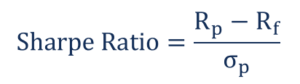Where:

Rp = Expected portfolio return

Rf = Risk free rate

Sigma(p) = Standard Deviation

### Risk-Adjusted Return Ratios – Treynor Ratio

The Treynor ratio measures the return earned in excess of what could’ve been earned if the asset did not have any diversifiable risk. It uses a beta coefficient instead of a standard deviation. Created by American economist Jack L Treynor, the ratio shows how much return an investment can provide its investor while considering its inherent level of risk.

The beta coefficient depicts the sensitivity of the investment in the market. If the ratio shows a high value, it means the investment offers a relatively high return with the inclusion of market risks.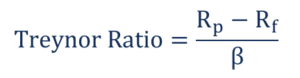Where:

Rp = Expected Portfolio return

Rf = Risk free rate

Beta(p) = Portfolio Beta

#### Example (Treynor Ratio)

Suppose the five-year annual return for the DJIA is 18% and the average return on T-bills is 7%.

 Investment Average Annual Return Standard Deviation A 12% 0.92 B 10% 0.26 C 8% 0.20

Market = (0.18-0.07)/1= 0.11

A = (0.12-0.07)/0.92 = 0.05

B = (0.10-0.07)/0.26 = 0.11

C = (0.08-0.07)/0.20 = 0.05

### Risk-Adjusted Return Ratios – Jensen’s Alpha

Jensen’s Alpha is used to describe the active return on an investment. It measures the performance of an index against a market index benchmark and represents the market movement as a whole. The alpha shows the performance of the investment after its risk is considered.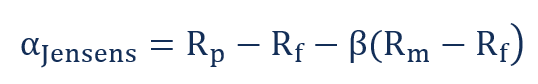Where:

Rp = Expected Portfolio return

Rf = Risk free rate

Beta(p) = Portfolio Beta

Rm = Market Return

#### Interpretation (Jensen’s Alpha)

Alpha < 0 means the investment was too risky for a return.

Alpha = 0 means the return earned is sufficient for the risk taken.

Alpha > 0 means the return earned is greater than the assumed risk.

#### Example (Jensen’s Alpha)

Assume a portfolio realized a return of 17% in the previous year. The market index returned 12.5%. The beta is 1.4, and the risk-free rate is 4%.

Jensen’s Alpha = 17- [4+1.4*(12.5-4)]=17-(4+11.9) = 1.1%

An alpha of 1.1% means the investor receives a high return for the risk assumed over the year.

### Risk-Adjusted Return Ratios – R-Squared

The R-Squared measures the percentage of a fund’s movements based on the movement of the benchmarked index. The ratio’s values can vary from 0%-100%. An R-Squared value of 100% means the movements of the index are justified by movements of the benchmarked index.

### Risk-Adjusted Return Ratios – Sortino Ratio

The Sortino ratio is a variation of the Sharpe ratio. It takes a portfolio’s return and divides it by the “Downside Risk.” Downside risk is the volatility of a portfolio’s return below a certain level. The level is based on average returns. The ratio measures the downside risk of a fund or stock. Like the Sharpe ratio, higher values indicate less risk relative to return.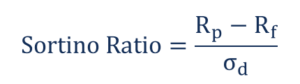Where:

Rp = Expected Portfolio return

Rf = Risk free rate

Sigma(d) = Standard deviation of a negative asset return

#### Example (Sortino Ratio)

A mutual fund shows an annual return of 16% and downside deviation of 9%. The risk-free rate is 3%.

Sortino = (16%-3%)/9% = 1.44

### Risk-Adjusted Return Ratios – Modigliani-Modigliani Ratio

The Modigliani-Modigliani ratio, also known as the M2 ratio, is used to derive the risk-djusted return of an investment. It shows the return on an investment adjusted for risk in comparison to a benchmark. It is shown as units of percentage return.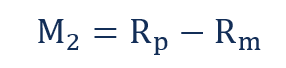Where:

Rp = return on adjusted portfolio

Rm = return on market portfolio

The adjusted portfolio is portfolio adjusted to show the total risk as the market portfolio.

#### Example (Modigliani-Modigliani Ratio)

Mutual Fund A shows an adjusted annual return of 15%, and the market index for the fund is 10%.

M2 = 15%-10% = 5%

### The Importance of Risk-Adjusted Returns

Risk is the divergence from an expected outcome. It can be expressed as an absolute form as it relates to a market benchmark and can either be positive or negative. If an investor wants to achieve large returns on an investment in the long term, they need to be open to incurring losses in the short term. The level of volatility depends on the risk tolerance of the investor.

Risk-adjusted return measures how much risk is associated with producing a certain return. The concept is used to measure the returns of different investments with different levels of risk against a benchmark. If an asset shows a lower risk than the overall market, any return on the asset above the risk-free rate will be considered a gain.

Risk adjusted return metrics offer the following benefits:

• Allows you to compare the risk between two or more investments
• Examines the changes in the risk-free rate that is not done by other risk-return ratios
• You can compare actual returns with those of a benchmarked index

### Conclusion

The risk-adjusted return can vary from one investment to another, as many external factors affect the level of risk. They include the availability of funds, risk tolerance, and the ability to hold a position for a long time in a volatile market.

An investor can improve risk-adjusted returns by adjusting their stock position by the volatility in the market.

### More Resources

Thank you for reading CFI’s guide to risk-adjusted return ratios. CFI is the official provider of the Financial Modeling and Valuation Analyst (FMVA)™ certification program, designed to transform anyone into a world-class financial analyst.

To keep learning and developing your knowledge of financial analysis, we highly recommend the additional resources below:

• Corporate Finance Ratios
• Risk Management
• Systematic Risk
• Systemic Risk

### Financial Analyst Training

Get world-class financial training with CFI’s online certified financial analyst training program!

Gain the confidence you need to move up the ladder in a high powered corporate finance career path.

Learn financial modeling and valuation in Excel the easy way, with step-by-step training.